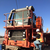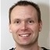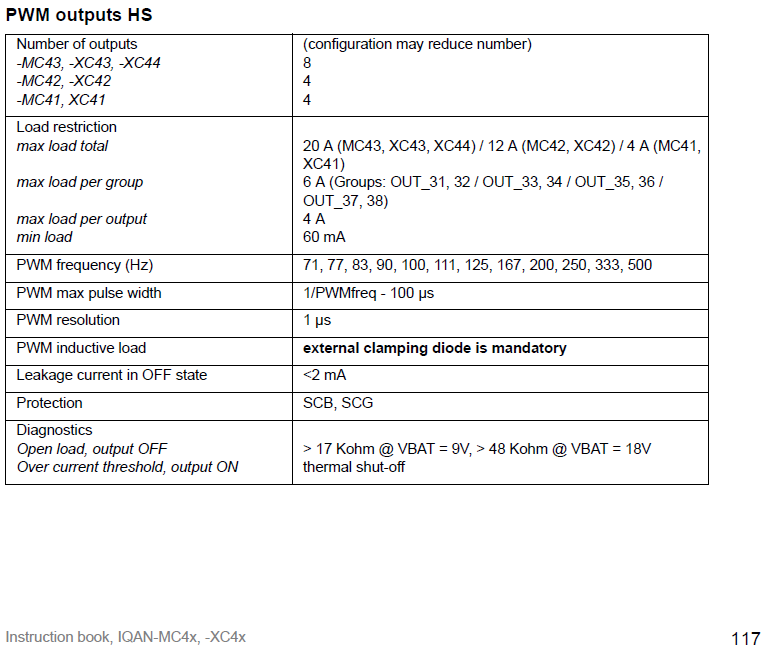0

# PWM MAX MR (Modulation Ratio)?

Ethan Zeman 4 years ago updated by Gustav Widén (System support) 4 years ago

The valid range for the PWM Max MR is 100.  Does this mean the PWM be 100% on and 0% off (essentially a digital out)?  or is this a percentage value applied to the actual max (i.e. MAX MR = 100 is equivalent to a PWM wave form that is 95% on 5% off)?The PWM out has a maximum pulse width that differs between the different modules.

So even with the PMW out set to 100, you still have a PWM.

I assume the question is about MC4x / XC4x modules?

You find the max pulse width in the Appendix A info:https://www.parker.com/Literature/Electronic%20Controls%20Division/Literature%20files/IQAN-MC4x_XC4x_MSG17-8413-IB_UK.pdf

Example:

If your PWM frequency is 100 Hz, the MC4x will give 99% pulse with

Period time = 1/100 = 10 ms

Max pulse with = 1/100 -100 μs  = 10 ms - 100 μs = 9.9 ms

Max MR% = 9.9 ms /10 ms = 99%